# The Medical College Admission Test (MCAT)

## Physical Sciences

1. C. The tangential (linear) velocity of an object moving around a circular path (velocity tangent to the circle) is proportional to the angular velocity.
v = r w, where r is the radius (9 m in this case) and w is the angular velocity (which must be in radians/sec for use in this formula).
2. D. A “g” force is equal to the actual weight, mg, of a person or object, so:
8 “g's” = 8(82 kg)(9.8 m/sec2) = 6429 N.
3. D. The centrifugal force is the “equal and opposite force” of Newton's third law and thus does NOT act on the pilot. It is the force that the pilot exerts on the back of his seat.
4. A. Power in watts is the rate of energy use, thus the energy, E = P × t or E = 450 watts × 120 sec = 54,000 joules and liters of O2 consumed = 54,000 J/(20,000 J/L) = 2.7 L.
5. D. According to Newton's third law, the pilot exerts a force of 8 “g's” on his seat back. Notice that this is the centrifugal force and it is a real outward force on the seat back. (The pilot, in his rotating “frame of reference,” feels as if a large outward force is pushing him into the seat back. This force is a “fictitous” force because the only real force acting on him is the inward centripetal force.)
6. D. The necessary inward-pointing centripetal force is unchanged. Nothing has changed except the device that supplies the centripetal force.
7. B. It is given in the passage that the telescope length is approximately equal to the sum of the focal lengths. If the total length is 4 m, then the value of fe is 4 m − fo = 1 m.
8. B. The objective lens forms a real, inverted image. The eyepiece acts in the same way that a magnifying glass does. The observer looks through the eyepiece and sees a final, virtual image that has the same orientation as the first image (that is, the final image is still inverted). This can be corrected by inserting a third lens, known as an erecting lens, between the objective and eyepiece.
9. C. The magnification of a simple Newtonian telescope is the ratio of the objective focal length to the eyepiece focal length:
M = fo/fe = 3/1 = 3 X.
10. B. This question requires an understanding of total internal reflection. The critical angle is the incident angle for which Snell's law of refraction predicts a refracted angle of 90°, for light rays traveling from a medium of larger index of refraction toward a medium of lower index of refraction. Here the light ray is incident inside the glass prism toward air of index, nair = 1. Using Snell's law:
nglass sin Øc = nair sin 90°, and because nglass = 1.52; sin Øc = 1/1.52 and Øc = 41°.
11. D. The objective lens is a projection lens, producing an inverted real image. The first image lies within the focal length of the eyepiece, which therefore acts as a magnifying glass, forming a virtual, enlarged image. The eyepiece does not invert the image.
12. B. As the object (primate) moves closer to the objective, the real image formed by the objective is further away from the objective, i.e., closer to the original position of the eyepiece. To see the image clearly, the eyepiece must be moved “back” away from the objective in order to focus the second (virtual) image clearly. (It is the eye-piece that is adjustable in telescopes and binoculars.) This is similar to holding this printed page too close to your eyes to see clearly. If you hold the page still and move your head away you will be able to see the print clearly.
13. B. The pressure is due to the weight of a column of fluid 75 m tall. P = dgy; where d = density of the liquid, and y is the depth below the surface; g is the acceleration of gravity, 9.8 m/sec2.
14. C. This is a simple conversion problem: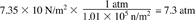15. C. Pressure is force divided by area, or P = F/A; so F = PA. It is necessary to convert the area from cm2 to m2 (by multiplying by 10−4m2/cm2). Thus F = 6.86 × 105 N/m2 × 65 cm2 × 1 × 10−4m2/cm2 = 4460 N.
16. A. Treating the air as an ideal gas, we see that P1 V1 = P2 V2, or V2 = P1V1/P2. Notice that we must use the absolute pressures in this expression. Then P1 = 8.36 × 105 N/m2 and P2 = 1.01 × 105 N/m2.
17. C. The partial pressure is the same fraction (0.21) of the total absolute pressure of 8.36 × 105 N/m2 as the fraction of oxygen by volume in ordinary air.
18. C. The work done on charged particles by electric fields depends on the charge of the particle and the voltage difference (not on the mass of the particle). The electric work, W = qV, increases the kinetic energy of the particles, in this case from zero to the final KE. Because the charges for electron and proton are equal, they have equal final kinetic energies (although the electron has a greater speed).
19. C. We need to know the force (F = qvB sinθ) on a moving charge in a magnetic field and we need the “right-hand rule” for the vector direction of the force on a positively charged particle (knowing the vector velocity and vector magnetic field, B). For a positive charge moving horizontally north through a magnetic field pointing horizontally west, the magnetic force is vertically upward. The negative electron is equivalent to a positive charge moving in the opposite direction so the force on both electron and proton is upward.
20. A. The magnetic force acts only at right angles to the velocity of a charged particle. It can change the direction of the velocity vector but not its magnitude (speed is the magnitude of velocity).
21. C. The charged particles are now moving parallel to the magnetic field direction so that sin θ is zero in the magnetic force equation (F = qvB sin θ). The magnetic force on each is zero, and their paths are not deflected.
22. B. The electric force, F = qE, shows that the direction of the force is parallel to E for positive charges like protons, and opposite (antiparallel) for negative charges like electrons.
23. B. The speed of any wave is the product of its frequency and wavelength, v = fλ. This is true for all waves, including sound, light, radio waves, and so forth. Thus λ = v/f.
24. A. The angular acceleration is the change in angular velocity divided by the time taken, or α = (ωf − ωi)/t. Converting ω to rad/sec: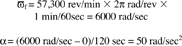25. B. Under the conservation of energy principle, the initial kinetic energy will be entirely converted into work done by friction in stopping the block. (The energy is dissipated as frictional heat.)26. B. A lens with a negative focal length is a diverging lens. The power in Diopters is the reciprocal of the focal length in meters; thus
P = −1/0.5 = − 2.0 D.
27. B. The product of force and time, Ft, is the impulse and equals the change in momentum of an object, pfpi. The initial momentum of both masses is zero. Since F and t are the same for both masses, their final momenta must be the same, so 2 N × 4 sec = 8 kg-m/sec. (Note that the 3 kg mass is moving faster but has the same momentum as the 6 kg mass.
28. C. Gamma (γ) emission is the emission of a photon from an excited nucleus and will change neither the charge nor mass number. The resulting nuclide is the (unexcited) Cu64. Emission of a negative electron, β, from the nucleus leaves it with one additional unit of positive charge so that the atomic number, z, increases by 1. The result is 30Zn64. Emission of a positron, β+, leaves the nucleus with one less positive charge and z decreases by 1. The result is 28Ni64. Note that the mass changes are so small for beta decays that the superscript mass number (64) remains the same in all three cases.
29. A. The index of refraction is n = c/v, where c is the speed of light in a vacuum (3 × 108 m/sec) and v is the speed of light in the material. Here, vs = 0.83 vw. Or vs = 0.83 c/nw = c/ns. Cancel c and solve for ns = 1.6.
30. B. The Coulomb force law between charged particles depends inversely on the square of the distance between the particles. F = kq1 q2/r2. Here the force increases by a factor of 4 because r2 is one-half of r1.
31. A. Newton's second law states that the net force equals the product of mass and acceleration: Fnet = ma. In this case the net force is the difference between the applied force (18 N) and the force of friction (9 N): Fnet = FaFf = 18 − 9 = (90)a.
32. B. The total momentum of the two-cart system is zero before the students push off, and remains zero after they separate. Then: 0 = m1v1 + m2v2. Thus, v2 = 60 kg(1.2 m/sec)/(0.8 m/sec).
33. B. The initial kinetic energy is used up in doing work against the frictional force as the student-cart is brought to rest. Using the conservation of energy principle: Ffx = 1/2 mv2, where x = 8 m. Then: Ff = 20 N.
34. C. We know that the total angular momentum of the student is conserved (constant). Because he is capable of changing his moment of inertia about the vertical axis of spin by extending his arms or pulling them in, we can find the ratio of the moments of inertia by using the conservation law in the form: I1 Ω1 = I2 Ω2. Then I2/I1 = Ω12 = 1.5.
35. D. Linear momentum is conserved in this problem. The addition of mass causes a decrease in the velocity as given by: m1v1 = (m1 + msand) V2. V2 = 1.6 m/sec.
36. B. The center-of-mass will not move because the forces exerted by pulling on the rope are internal forces to the two-cart system. It is nearer the larger mass, and is found by using the formula for the cm: Xcm = (m1x1 + m2/x2)/(m1 + m2). We are allowed to place the origin of the coordinate system anywhere we choose. Placing the origin at the original position of the larger mass, x1 = 0 and x2 = 2 m. Xcm = (0 + (80)(2))/(200 kg) = 0.8 m.
37. C. Conservation of momentum applies to this completely inelastic collision as follows: m1v1 = (m1 + m2)V. Then V = (80 kg)(4 m/sec)(80 + 80) = 2 m/sec.
38. B. The net force accelerates the 100 kg mass according to Newton's Third Law. The net force here is the difference between the applied 90 N force and the opposing friction force. Fnet = 90 − f = ma = 100 kg × 0.8 m/sec2. Solving for f we find that f = 10 N.
39. B. The falling weight can be isolated so that we can apply Newton's third law to it alone. The weight force due to the mass acts downward and the 90 N tension upward. The third law then shows that: mg − 90 = ma, which we solve for the unknown mass, m. m(ga) = 90 N. m(9.8 − 0.8) = 90. Then m = 10 kg.
40. C. The work done in accelerating the cart is equal to the gain in kinetic energy: Fnetx=1/2 mv2, or (100 N)(10 m) = (1/2) 80v2 and then v = 5 m/sec.
41. C. Both [H+] and [OH] = 1.0 × 10−7. The pH = 7.0.
42. B.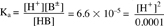Because the square root of 66 is about 8, [H+] = 8 × 10−5 or between 1 × 10−4 and 1 × 10−5 pH = − log [H+] and is between 4 and 5. Although water itself contributes [H+] = 1 × 10−7, this is negligibly small in comparison to the H+ from benzoic acid. Thus, in this example, the H+ from water may be neglected.
43. D. pH + pOH = 14
Although in pure water pH = pOH, that is not the case here. Because pH is between 4 and 5, however, this pOH will be between 9 and 10.
44. B.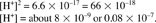This is very small compared with the [H+] of 1.0 × 10−7 supplied by ionization of water. Thus the pH approximates 7.
45. A. Henderson-Hasselbalch formulaWhen the concentrations of salt and acid are equal, pH = pKa. By inspection of Ka = 6.6 × 10−5, its negative log would be between 4 and 5. Thus the pKa and the resulting pH are between 4 and 5.
46. D.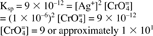47. C. Addition of Cl ions will bring about precipitation of AgCl when its Ksp is exceeded.
48. B. The Ksp is given for a particular temperature and will change with temperature. It may speed the reaction but will not likely bring about more complete precipitation.
49. C. The Ksp with the smallest number represents the one with the lowest solubility.
50. D. The Ksp's are independent of each other. If the Ksp of one is not reached, no precipitation will occur.
51. A. Phosphorus, a nonmetal, will react with oxygen to form an oxide. This oxide will react with water to form an acidic solution.
52. A. Nitrogen, a nonmetal, will react with oxygen to form an oxide. This oxide will react with water to form an acidic solution.
53. B. One mole of Ca will form one mole of CaO by oxidation. This will form one mole of Ca(OH)2 by reaction with water.
54. C. One mole of Ca(OH)2 will require two moles of HCl for neutralization.
55. A. Oxides of sulfur are produced during burning of coal. These acid anhydrides react with water to form acids. Carbon dioxide can react with water to form the weak acid, carbonic acid. There is already a substantial amount of carbon dioxide in the atmosphere. Although carbon dioxide above the earth may contribute to other problems, it has not been suggested to contribute to acid rain.
56. C. One mole of each would exert the same effect, but only if they are ionized. NaCl produces 2 ions per molecule, and Na2SO4 produces 3 ions per molecule. Colligative properties depend on the number of particles.
57. B. Molality (moles of solute per 1000 g of solvent) is used in calculations of colligative properties. Further calculations are required if there is ionization.
58. D. All of these are colligative properties.
59. A. Without knowing the identity of the solute, we know that twice the amount will lower the freezing point twice as much.
60. C. If the weight is the same, the nonionizing solute with the lowest molecular weight will have the largest number of dissolved molecules.
61. A. Loss of a single electron in the 3s orbital leaves the stable electronic configuration of the noble gas, neon.
62. B. In a neutral atom, the number of electrons in orbitals equals the number of protons in the nucleus. The latter defines the atomic number.
63. C. Gain of an additional electron would give the stable electronic configuration of the noble element, krypton.
64. D. Note that the number of electrons exceeds the atomic number (and the number of protons) by one. Thus, this would be an ion with a charge of −1.
65. C. The fact that the number of electrons is equal to the atomic number indicates that this is a neutral atom. The fact that the outermost electron is in the 3p orbital rather than the expected 3s orbital indicates that the atom is in an excited state.
66. D. Noble elements include helium, neon, argon, krypton, xenon, and randon.
67. D. Each orbital electron possesses four quantum numbers – principal, angular momentum, magnetic, and spin.
68. C. Possible values of magnetic quantum number are −1, 0, and +1.
69. B. Possible values of spin quantum number are70. A. No two electrons in an atom possess all four identical quantum numbers.
71. C. Silver ions will react with chloride ions and precipitate as AgCl.
72. B. H2S ⇄ 2H+ + S2−. By the common ion effect, lowering the pH (increasing the H+ concentration) will lower the S2− concentration by displacing the reaction to the left.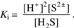If [H+] increases, [S2−] must decrease.
73. A. Freezing point depression in water depends only on the number of solute particles per unit volume
1 M NaCl = 2 × 1 × 6.02 × 1023 particles per liter
0.3 M Na2SO4 = 3 × .3 × 6.02 × 1023 particles per liter
1.5 M glucose = 1 × 1.5 × 6.02 × 1023 particles per liter
0.5 M BaSO4 = 2 × 0.5 × 6.02 × 1023 particles per liter
Dividing by 6.02 × 1023 we can see that the comparative figures are NaCl, 2; Na2SO4, 0.9; glucose, 1.5; and BaSO4, 1.0. Thus, the NaCl solution has the greatest number of particles per unit volume (considering the ionization of NaCl, Na2SO4, and BaSO4), and it will have the lowest freezing point.
74. D. A reaction in which electrons (e) are removed is termed an oxidation reaction; the adding of electrons to an atom or molecule is termed a reduction reaction.
75. A. The hydronium ion, H3O+, is a protonated water molecule. 2H2O ⇄ H3O+ + OH
76. A. As long as the volume units are the same, N1V1 = N2V277. A. This is a definition of the zwitterion; an example is the amino acid, glycine.